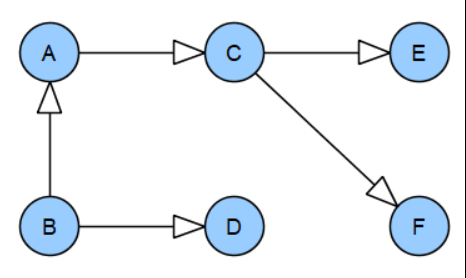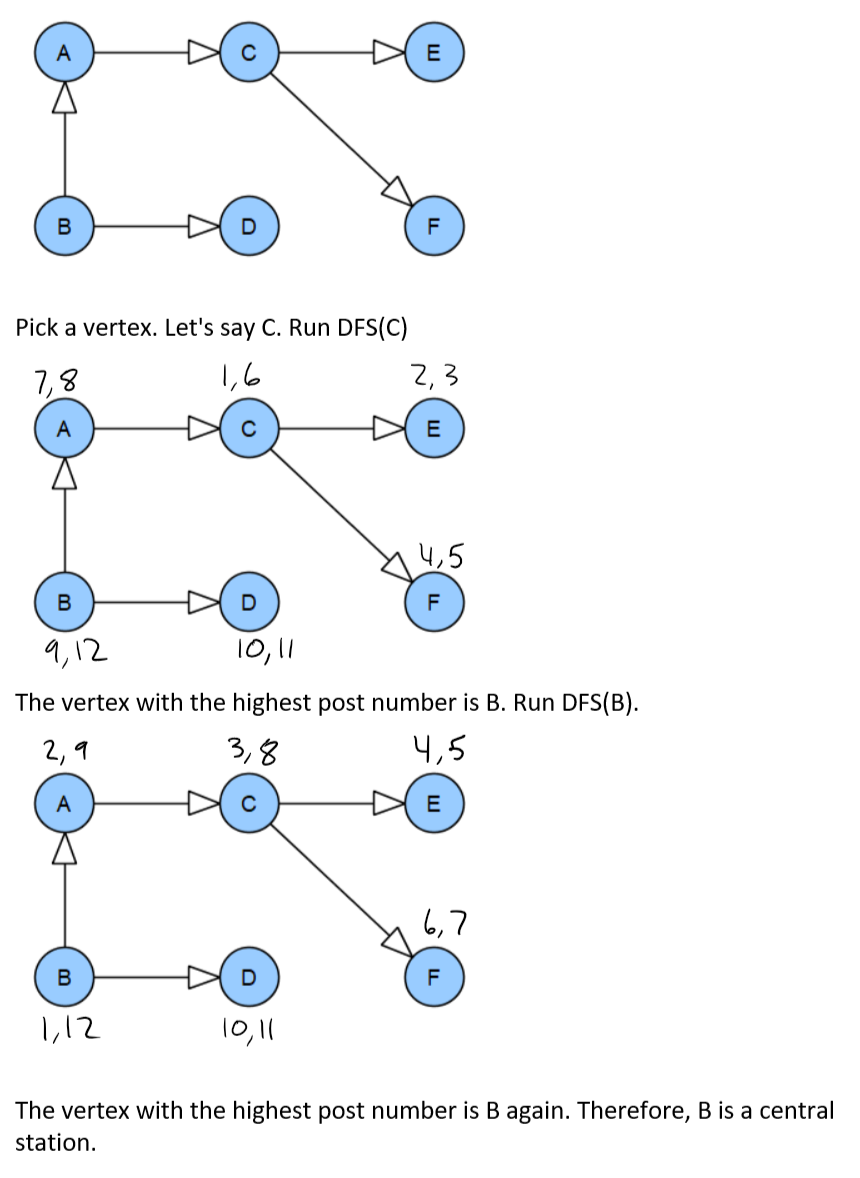# Graph Class¶

The directed graph will be implemented with an adjacency map. The adjacency map is a dictionary of vertex labels pointing to vertices.

Each vertex stores a list of out-edges. An "out-edge" is an edge starting at a particular vertex and pointing out to a different vertex.

In :
class Vertex:

def __init__(self, label):
self.label = label
self.edges = []
# Used to track if this vertex has been visited during depth-first search.
self.visited = False

'''Adds an edge with optional weighting. Weights ended up not being relevant for this notebook.'''
self.edges.append((destinationVertex, weight))

def __repr__(self):
return f"{self.label}"

def __eq__(self, other):
return self.label == other.label

In :
class Graph:

def __init__(self):
self.vertices = {}

newVertex = Vertex(vertexLabel)
self.vertices[vertexLabel] = newVertex

sourceVertex = self.vertices[sourceVertexLabel]
destinationVertex = self.vertices[destinationVertexLabel]


## Test Graph¶In :
G = Graph()


## Vertices¶

In :
labels = "A, B, C, D, E, F".split(",")
labels

Out:
['A', ' B', ' C', ' D', ' E', ' F']
In :
for label in labels:
# strip white space from each letter e.g. " B" -> "B"

In :
G.vertices

Out:
{'A': A, 'B': B, 'C': C, 'D': D, 'E': E, 'F': F}

## Edges¶

In :
edges = [
("A", "C"),
("B", "A"),
("B", "D"),
("D", "C"),
("C", "E"),
("C", "F")
]

In :
for edge in edges:

In :
G.vertices['A'].edges

Out:
[(C, 1)]

# Finding a Central Station¶

## What is a Central Station?¶

A central station is a vertex that can reach all other vertices in the graph.

## Algorithm¶

Basically:

1. Pick a random vertex.
2. Perform DFS. Give each vertex a pre and post number.
3. Run DFS again, this time from the vertex with the largest post number. If this vertex can reach all other vertices, it is a central station. If it cannot, the graph has no central station.

## Implementation¶

As far as the implementation is concerned, there is no real point to labeling each vertex with pre and post numbers. The last node visited will be the node with the highest post number and since this is the only vertex we care about numbering is unnecessary.

In :
def explore(vertex, count):
'''Recursive DFS generator used to explore one vertex.'''
vertex.visited = True
# previsit
count = count + 1
yield ("previsit", count, vertex)

for edge in vertex.edges:
child = edge
if not child.visited:
# visit children
yield from explore(child, count)

# postvisit
count = count + 1
yield ("postvisit", count, vertex)

In :
def dfs(graph, vertexLabel):
'''DFS generator that yields a tuple of data for each vertex
(pre and post visit). The tuple is:
('previsit' or 'postvisit', count, vertex).'''

# For pre and post numbering. Not really needed, but could be helpful to see this.
# Using a list as a hack to keep count mutable.
count = 

# Set all vertices to unvisited.
for vertex in graph.vertices.values():
vertex.visited = False

# Get the starting vertex.
startingVertex = graph.vertices[vertexLabel]

# Explore the starting vertex.
yield from explore(startingVertex, count)

# If there are vertices in the graph that are not connected to the starting
# vertex, they will be missed. Check for this.
for vertex in graph.vertices.values():
if not vertex.visited:
# This vertex hasn't been explored yet.
yield from explore(vertex, count)

In :
for v in dfs(G, 'C'):
print(v)

('previsit', 1, C)
('previsit', 2, E)
('postvisit', 3, E)
('previsit', 4, F)
('postvisit', 5, F)
('postvisit', 6, C)
('previsit', 7, A)
('postvisit', 8, A)
('previsit', 9, B)
('previsit', 10, D)
('postvisit', 11, D)
('postvisit', 12, B)


The vertex with the highest postnumber could be a central station. To ensure that it is, run DFS again from this vertex.

In :
for v in dfs(G, 'B'):
print(v)

('previsit', 1, B)
('previsit', 2, A)
('previsit', 3, C)
('previsit', 4, E)
('postvisit', 5, E)
('previsit', 6, F)
('postvisit', 7, F)
('postvisit', 8, C)
('postvisit', 9, A)
('previsit', 10, D)
('postvisit', 11, D)
('postvisit', 12, B)


Since B has the highest post number again, it is a central station.

Wrapping this up into a function:

In :
def centralStation(graph):
startingVertex = next(iter(graph.vertices.values()))
numberOfVertices = len(graph.vertices.values())

# First DFS
for visit in dfs(graph, startingVertex.label):
count = visit
vertex = visit
if count == numberOfVertices * 2:
highestPostVertex1 = vertex

# Second DFS
for visit in dfs(graph, highestPostVertex1.label):
count = visit
vertex = visit
if count == numberOfVertices * 2:
highestPostVertex2 = vertex

if highestPostVertex1.label == highestPostVertex2.label:
return highestPostVertex1

# No central station.
return None

In :
centralStation(G)

Out:
B

By hand: